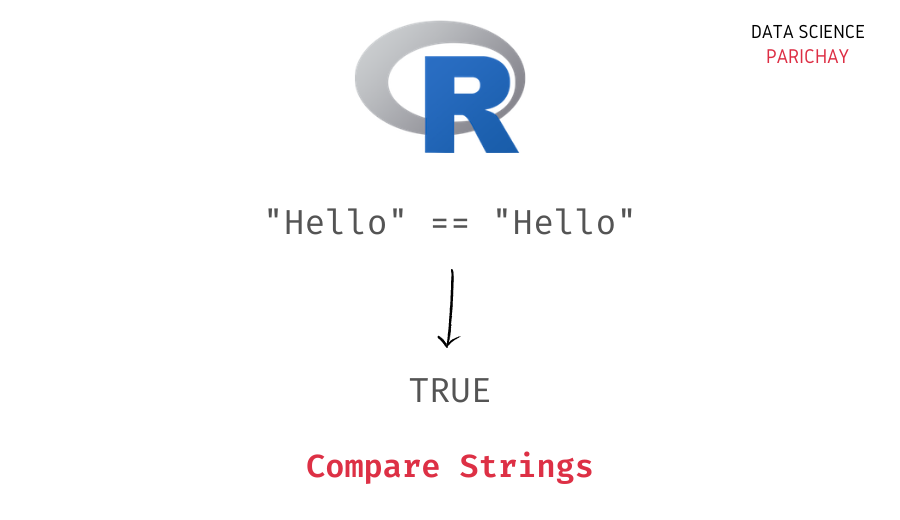# Compare Two Strings in R (With Examples)

In this tutorial, we will look at how to compare two strings for equality in R with the help of some examples.

## How do you compare two strings in R?

You can use the equality operator `==` to compare two strings in R. It performs a case-sensitive equality check. The following is the syntax –

```# compare string s1 and s2 for equality
s1 == s2```

It returns `TRUE` if both strings are equal and `FALSE` if they are not equal.

To compare strings irrespective of their case, either convert both the strings to lowercase or uppercase before checking for equality.

## Examples

Let’s now look at some examples of comparing two strings in R.

### 1. Compare two strings for equality

Let’s create some strings and compare them for equality using the `==` operator.

```# create strings
s1 <- "Hello"
s2 <- "Hello"
s3 <- "hello"

# compare strings
print(s1 == s2)
print(s1 == s3)```

Output:

``` TRUE
 FALSE```

Here, we create three strings s1, s2, and s3. We get `TRUE` for `s1 == s2` because both the strings are the same. Whereas we get `FALSE` for `s1 == s3`, this is because even though both strings contain the same alphabets, the cases are different and thus in a case-sensitive check for equality both strings are considered unequal.

📚 Data Science Programs By Skill Level

Introductory

Intermediate ⭐⭐⭐

🔎 Find Data Science Programs 👨‍💻 111,889 already enrolled

Disclaimer: Data Science Parichay is reader supported. When you purchase a course through a link on this site, we may earn a small commission at no additional cost to you. Earned commissions help support this website and its team of writers.

### 2. Compare two strings for equality irrespective of the case

To compare strings irrespective of their case, either convert both the strings to lowercase or uppercase before checking for equality using the `==` operator. This will mitigate any differences arising due to differences in case.

Let’s look at an example.

```# create strings
s1 <- "Hello"
s2 <- "Hello"
s3 <- "hello"

# compare strings
print(tolower(s1) == tolower(s2))
print(tolower(s1) == tolower(s3))```

Output:

``` TRUE
 TRUE```

Here, we use the same strings as above, s1, s2, and s3 but this time we first convert each string to lowercase using the R `tolower()` function before comparing them for equality.

We get `TRUE` for both `s1 == s2` and `s1 == s3`.

You might also be interested in –

•